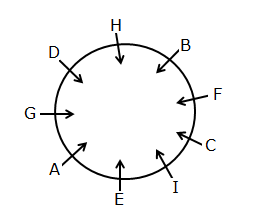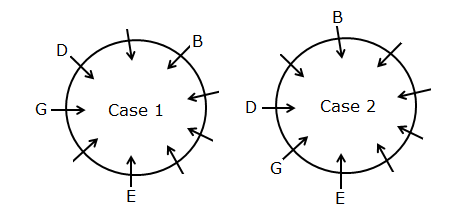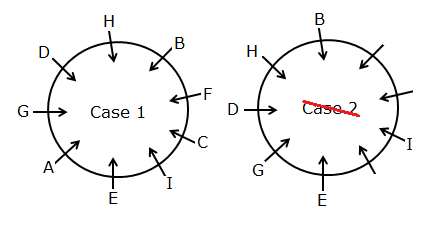# RRB Clerk Prelims Reasoning Ability Questions 2021 (Day-09)

Dear Aspirants, Our IBPS Guide team is providing new series of Reasoning Questions for RRB Clerk Prelims 2021 so the aspirants can practice it on a daily basis. These questions are framed by our skilled experts after understanding your needs thoroughly. Aspirants can practice these new series questions daily to familiarize with the exact exam pattern and make your preparation effective.

Start Quiz

Circular arrangement

Direction (1-5): Answer the questions based on the information given below.

Nine persons viz. A, B, C, D, E, F, G, H and I are standing on a circular line such that all of them are facing towards the center, but not necessarily in the same order. The consecutive alphabetically named persons don’t stand adjacent to each other.

E stands four persons away from B who stands second to the left of D. G stands third to the right of B. H stands fourth to the right of I. A stands third to the left of C.

1) Who among the following person stands to the immediate left of B?

A.H

B.C

C.I

D.A

E.None of these

2) What is the position of D with respect to A?

A.Second to the right

B.Third to the right

C.Second to the left

D.Third to the left

E.None of these

3) How many persons are there between H and E when counted from the right of E?

A.1

B.2

C.3

D.More than three

E.None

4) If all the persons are made to stand in alphabetical order in a clockwise direction with respect to A, then how many persons remain unchanged in their position, excluding A?

A.1

B.2

C.3

D.More than three

E.None

5) Who among the following person stands fourth to the left of I?

A.B

B.A

C.D

D.G

E.H

Inequality

Direction (6-9): In each of the following questions, the relationship between different elements is shown in the statements followed by two conclusions. Find the conclusion which is definitely true.

6) Statements:

A > D > R < T = F ≤ M < S > G

Conclusions:

I).  A > T

II). R < S

A.If only conclusion I is true.

B.If only conclusion II is true.

C.If either conclusion I or II is true.

D.If neither conclusion I nor II is true.

E.If both conclusions I and II are true.

7) Statements:

N > Y > R = W > S ≤ V ≥ L = H

Conclusions:

I). N > S

II). H ≥ V

A.If only conclusion I is true.

B.If only conclusion II is true.

C.If either conclusion I or II is true.

D.If neither conclusion I nor II is true.

E.If both conclusions I and II are true.

8) Statements:

X > Y = Z ≥ Q ≥ R ≥ I = P > N

Conclusions:

I). Y > N

II). Z < P

A.If only conclusion I is true.

B.If only conclusion II is true.

C.If either conclusion I or II is true.

D.If neither conclusion I nor II is true.

E.If both conclusions I and II are true.

9) Statements:

S > R > E < H ≥ W ≥ T > A > M

Conclusions:

I). S < M

II). E > A

A.If only conclusion I is true.

B.If only conclusion II is true.

C.If either conclusion I or II is true.

D.If neither conclusion I nor II is true.

E.If both conclusions I and II are true.

Misc

10) If in the number 246357285, the digits at the odd position are increased by 1 and the digits at the even position are decreased by 2 from the left end, then in the number thus obtained how many digits are even numbers?

A.Two

B.Three

C.More than three

D.One

E.None

:

Directions (1-5):1) E stands four persons away from B who stands second to the left of D. G stands third to the right of B.2) H stands fourth to the right of I. A stands third to the left of C. Hence, case 2 gets eliminated.